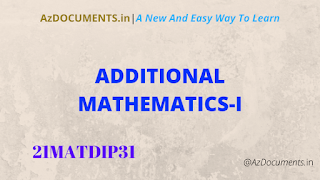##A bridge course for Lateral Entry Students under Diploma quota to BE/B.Tech. programme

### Module-1

Differential Calculus: Successive differentiation-problems. Taylor’s & Maclaurin’s series expansions-problems. Partial Differentiation: Euler’s theorem (without Proof)-problems on first order derivatives only. Total derivatives-differentiation of composite functions. Jacobians of order two-Problems.(RBT Levels: L1, L2 and L3) 8 Hours

### Module-2

Complex Numbers: Definitions and properties. Modulus and amplitude of a complex number, Argand’s diagram, De-Moivre’s theorem (without proof), Problems. Vector Algebra: Scalar and vectors. Addition, subtraction and multiplication of vectors- Dot and Cross products, problems. Scalar triple product, Problems. 8 Hours (RBT Levels: L1, L2 and L3) (RBT Levels: L1 and L2)

### Module-3

Vector Differentiation: Differentiation of vector functions. Velocity and acceleration of a particle moving on a space curve. Scalar and vector point functions. Gradient, Divergence, Curl-simple problems. Solenoidal and irrotational vector fields-Problems. 8 Hours (RBT Levels: L1, L2 and L3)

### Module-4

Integral Calculus: Review of elementary integral calculus. Reduction formulae for sin𝑛 𝑥 , cos𝑛 𝑥, sin𝑛 𝑥 cos (without proof) and evaluation of these with standard limits-problems. Double and triple integrals-Simple problems. 8 Hours (RBT Levels: L1, L2 and L3)

### Module-5

Ordinary Differential Equations (ODEs): Introduction-solutions of first order and first-degree differential equations: Variable separable method, Homogeneous differential equations, linear differential equations. Exact differential equations. 8 Hours (RBT Levels: L1 L2 and L3)

Suggested Learning Resources:

Books

1. Higher Engineering Mathematics, B. S. Grewal, Khanna Publishers, 43rd Edition, 2015.

2. Advanced Engineering Mathematics, E. Kreyszig John, Wiley & Sons, 10th Edition, 2015.

3. Engineering Mathematics, N. P. Bali and Manish Goyal, Laxmi Publishers, 7th Edition, 2007.

4. Higher Engineering Mathematics, H. K. Das and Er. Rajnish Verma, S. Chand & Company